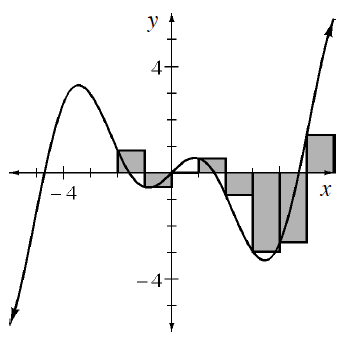### Home > APCALC > Chapter 2 > Lesson 2.2.2 > Problem2-69

2-69.

Using sigma notation, write a Riemann sum to estimate the area under the function $f(x)=x\cos(x)$ for $−2\le x\le6$ with eight left endpoint rectangles of equal width. Then use the summation feature of your graphing calculator to calculate the estimated area.

Since $\text{area of a rectangle} = \left(\text{base}\right)\left(\text{height}\right)$, start by finding the base, or $\Delta x$ of each rectangle. Divide the interval by the number of rectangles:

$\Delta x=\frac{6-(-2)}{8}=1$

Next, find the height of each rectangle. The height is determined by $f(a +\Delta xi)$, where $a = \text{starting value on the }x\text{-axis}$.
$f((−2) + (1)i) = f(−2 + i)$

Finally, use sigma notation to represent the sum of all eight rectangles. Since these are left-endpoint rectangles, the index will start at $0$ and end at $n − 1$, where $n = \text{number of rectangles}$.

$\displaystyle \sum_{i=0}^{n-1}\Delta xf(a+\Delta xi)=\sum_{i=0}^{7}(1)f(-2+i)=\sum_{i=0}^{7}f(-2+i)$

Use your calculator to evaluate this sum.